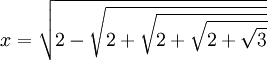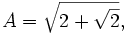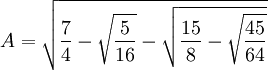## Sunday, January 21, 2007

### Solving Van Roomen's Problem: Step Five

In today's blog, I show how we can use the trigonometric identities in my previous blog to simplify Van Roomen's x and A examples. To see the statement of Van Roomen's problem, start here.

The content in today's blog is taken from Jean-Pierre Tignol's Galois' Theory of Algebraic Equations.

Lemma 1:= 2 sin(15π/25)

Proof:

(1) cos(90°) = 0 [See Property 7, here]

(2) cos(2π/4) = cos(π/2) = cos(90°) = 0 [See here for review of radians]

(3) 2cos(π/4) = ±√2 + 2cos(π/2) [See Lemma 1, here]

(4) So, 2cos(π/4) = ±√2

(5) 2cos(π/8) = ±√2 + 2cos(π/4) = ±√2 + √2

(6) 2cos(π/16) = ±√2 + 2cos(π/8) = ±√2 + √(2 + √2)

(7) 2cos(π/32) = ±√2 + 2cos(π/16) = ±√2 + √[2 + √(2 + √2)]

(8) 2cos(π/32) = 2sin(π/2 - π/32) [See Lemma 1, here]

(9) 2sin(π/2 - π/32) = 2sin(16π/32 - π/32) = 2sin(15π/32) = 2sin(15π/25)

QED

Lemma 2:= 2 sin(π/[25*3])

Proof:

(1) 2cos(π/6) = √3 [See Corollary 1.1, here]

(2) 2cos(π/12) = ±√2 + 2cos(π/6) [See Lemma 1, here]

(3) 2cos(π/12) = ±√2 + √3

(4) 2cos(π/24) = ±√2 + 2cos(π/12) = ±√2 + √(2 + √3)

(5) 2cos(π/48) = ±√2 + 2cos(π/24) = ±√2 + √[2 + √(2 + √3)]

(5) 2sin(π/96) = ±√2 - 2cos(π/48) [See Lemma 2, here]

(6) 2sin(π/96) = 2sin(π/[25*3]) = ±√2 - {2 + √[2 + √(2 + √3)]}

QED

Lemma 3:= 2 sin(15π/26)

Proof:

(1) From step#6 in Lemma 1 above, we have:

2cos(π/16) = ±√2 + √(2 + √2)

(2) Using Lemma 2 here, we have:

2sin(π/32) = ±√2 - 2cos(π/16) = ±√2 - √[2 + √(2 + √2)]

(3) 2cos(π/2 - π/32) = 2sin(π/32) [See Corollary 1.1, here]

(4) 2cos(π/2 - π/32) = 2cos(16π/32 - π/32) = 2cos(15π/32)

(5) 2sin(15π/64) = ±√2 - 2cos(π/16) = ±√2 - √{2 - √[2 + √(2 + √2)]}

(6) 2sin(15π/64) = 2sin(15π/)

QED

Lemma 4:= 2sin(π/[26*3])

Proof:

(1) Using step #5 from Lemma 2 above, we have:

2cos(π/24*3) = ±√2 + 2cos(π/24) = ±√2 + √[2 + √(2 + √3)]

(2) So we have:

2cos(π/25*3) = ±√2 + 2cos(π/24*3) = ±2 + {2 + √[2 + √(2 + √3)]}

(3) And also:

2sin(π/26*3) = ±√2 - 2cos(π/25*3) =

= ±
2 - √(2 + {2 + √[2 + √(2 + √3)]})

QED

Lemma 5:= 2sin(3π/23)

Proof:

(1) From Lemma 1 above, step #5, we have:

2cos(π/8) = ±√2 + 2cos(π/4) = ±√2 + √2

(3) Using Lemma 1 here we have:

2cos(π/8) = 2sin(π/2 - π/8) = 2sin(4π/8 - π/8) = 2sin(3π/8) = 2sin(3π/23)

QED

Lemma 6:= 2sin(π/[23*3*5])

Proof:

(1) π/5 - π/6 = 6π/30 - 5π/30 = π/30

(2) 2cos(π/30) = 2cos(π/5 - π/6) = 2cos(π/5)cos(π/6) + 2sin(π/5)sin(π/6) [See Theorem 2, here for cosine addition/subtraction rule]

(3) Using previous results, we can compute cos(π/10) and sin(π/10):

cos(π/10) = sin(π/2 - π/10) = sin(5π/10 - π/10) = sin(4π10) = sin(2π/5) [See here Lemma 1,here]

sin(2π/5) = (1/2)(5 + √5)/2 [See Lemma 6, here]

sin(π/10) = cos(π/2 - π/10) = cos(2π/10) [See Corollary 1.1, here]

cos(2π/5) = (√5 - 1)/4 [See Lemma 5, here]

(4) Now, we can use cos(π/10), sin(π/10) to compute cos(π/5) and sin(π/5):

Using the cosine double angle formula (see Corollary 3.1, here):
cos(2x) = 2cos2(x) - 1

So,
cos(2*π/10) = cos(π/5) = 2cos2(π/10) - 1 = (2)(1/8)[5 + √5] - 1 = 5/4 + (√5)/4 - 4/4 = (√5 + 1)/4

Now, we can use sin2(π/5) + cos2(π/5) = 1 [see Corollary 2, here], to get:

sin(π/5)2 = 1 - cos2(π/5) = 16/16 - (1/16)(5 + 2√5 + 1) = (1/16)[10 - 2√5 ]

This gives us:
sin(π/5) = (1/4)√10 - 2√5

(5) Using a previous result (see Corollary 1.2, here), we know that:

cos(π/6) = cos(30°) = √3/2

sin(π/6) = sin(30 °) = (1/2)

(6) So, this gives us that:

cos(π/30) = 2[(√5 + 1)/4][3/2] + 2[(1/4)√10 - 2√5][(1/2)] =

= (1/4)[15 + 3] + (1/4)[10 - 2√5] =(3/16) + (15/16) + (5/8) - √(5/64).

(7) 2cos(π/[22*5*3]) = ±√2 + 2cos(π/30) [See Lemma 1, here]

So that:
2cos(π/[22*5*3]) = ±√{2 + (3/16) + (15/16) + [(5/8) - √(5/64)]}

(8) 2sin(π/[23*5*3]) = ±√2 - 2cos(π/[22*5*3]) [See Lemma 2, here]

So:
2sin(π/[23*5*3]) = ±√2 - √{2 + (3/16) + (15/16) + [(5/8) - √(5/64)]}

QED

Lemma 7:= 2 sin(π/[3*5])

Proof:

(1) 2sin(π/15) = (1/2)√7 - √5 - √(30 - 6√5) [See Corollary 7.1, here]

(2) Since (1/2) = (1/√4), we have:

2sin(π/15) = √(7/4) - √(5/16) - √[(30 - √[36*5])/16] =

= √(7/4) - √(5/16) - √[15/8 - √(36*5/16*16)] =

= √(7/4) - √(5/16) - √[15/8 - √(9*5/4*16)] =

= √(7/4) - √(5/16) - √[15/8 - √(45/64)]

QED

References
• Jean-Pierre Tignol, Galois' Theory of Algebraic Equations, World Scientific, 2001

### Solving Van Roomen's Problem: Step Four

In today's blog, I will present some simple trigonometric identities that can be used to simplify Van Roomen's examples of x and A values. For those who want an introduction to Van Roomen's Problem, start here. In my next blog, I will apply these lemmas to Van Roomen's problem.

The content in today's blog is taken from Jean-Pierre Tignol's Galois' Theory of Algebraic Equations.

Let me start by introducting the some basic ideas from trigonometry.

Lemma 1: 2 cos(α/2) = ± √2 + 2 cos α

Proof:

(1) cos(2x) = 2 cos2(x) - 1 [See Corollary 3.1, here]

(2) Let x = α/2, then we get:

cos(α) = 2cos2(α/2) - 1

(3) Rearranging terms gives us:

2cos2(α/2) = cos(α) + 1

(4) Multiplying both sides by 2 gives us:

4cos2(α/2) = 2cos(α) + 2

(5) Finally, taking the square root of both sides gives us:

2cos(α/2) = ±√2 + 2cos(α)

QED

Lemma 2: 2 sin(α/2) = ± √2 - 2 cos α

Proof:

(1) cos(2x) = 1 - 2 sin2(x) [See Corollary 3.1, here]

(2) Let x = α/2, then we get:

cos(α) = 1 - 2sin2(α/2)

(3) Rearranging terms gives us:

2sin2(α/2) = 1 - cos(α)

(4) Multiplying both sides by 2 gives us:

4sin2(α/2) = 2 - 2cos(α)

(5) Finally, taking the square root of both sides gives us:

2sin(α/2) = ±√2 - 2cos(α)

QED

Lemma 3: 2 cos(π/3) = 1

Proof:

(1) 2cos(π/3) = 2cos(60°) [See here for review of radians]

(2) 2cos(60°) = 2(1/2) = 1 [See Corollary 1.2, here]

QED

Lemma 4: 2 sin(π/3) = √3

Proof:

(1) 2sin(π/3) = 2sin(60°) [See here for review of radians]

(2) 2sin(60°) = 2*(3/2) = 3 [See Corollary 1.2, here]

QED

Lemma 5: 2cos(2π/5) = (√5 - 1)/2

Proof:

(1) cos (2π/5) = (√5 - 1)/4 [See Corollary 1.1, here]

(2) So:

2cos(2π/5) = (√5 - 1)/2

QED

Lemma 6: 2sin(2π/5) = √(5 + √5)/2

Proof:

(1) sin (2π/5) = √5 + √5/8 [See Corollary 1.1, here]

(2) So:

2*sin (2π/5) = 2(1/2)√5 + √5/2 = √(5 + √5)/2

QED

Lemma 7: 2cos(π/15) = (1/4)(√30 + 6√5 + √5 - 1)

Proof:

(1) 2π/5 - π/3 = 6π/15 - 5π/15 = π/15

(2) 2cos(π/15) = 2cos(2π/5 - π/3) = 2cos(2π/5)cos(π/3) + 2sin(2π/5)sin(π/3) [See Theorem 2, here]

2cos(2π/5) = (√5 - 1)/2 [See Lemma 5 above]

cos(π/3) = 1/2 [See Lemma 3 above]

2sin(2π/5) = (5 + √5)/2 [See Lemma 6 above]

sin(π/3) = 3/2 [See Lemma 4 above]

(4) 2cos(π/15) = [ (√5 - 1)/2 ][1/2] + [(5 + √5)/2][3/2] =

= [ (√5 - 1)/4] +(1/2) [(15 + 3√5)/2] = [ (√5 - 1)/4] + (1/4)[(30 + 6√5)]

= (1/4)[5 - 1 + (30 + 6√5)]

QED

Corollary 7.1: 2sin(π/15) = (1/2)√7 - √5 - √(30 - 6√5)

Proof:

(1) 2cos(π/15) = (1/4)(√30 + 6√5 + √5 - 1) [See Lemma 7 above]

(2) 4cos2(π/15) = (1/16)(30 + 6√5 + 5 + 1 + 2(√30 + 6√5)(5) - 230 + 6√5 - 2√5 = (1/16)(36 + 4√5 + 2(√30 + 6√5)(5) - 230 + 6√5)

(3) Since sin2(x) + cos2(x) = 1 [See Corollary 2, here], we have:

4sin2(π/15) = 4 - cos2(π/15) =

= (1/16)[64 - 36 - 4√5 - 2(√30 + 6√5)(5) + 230 + 6√5 ] =

= (1/16)[28 - 4√5 - 2(√30 + 6√5)(5) + 230 + 6√5] =

= (1/16)[28
- 4√5 - (30 + 6√5)(25 - 2)] =

=
(1/16)[28 - 4√5 - (√30 + 6√5)(√2√5 - 2)2] =

= (1/16)[28 - 4√5 - (30 + 6√5)(√20 - 85 + 4)] =

=
(1/16)[28 - 4√5 - (30 + 6√5)(√24 - 85)] =

=
(1/16)[28 - 4√5 - (√720 - 240√5 + 1445 - 240)] =

= (1/16)[28 - 4√5 - (√480 - 96√5] =

= (1/16)[28 - 4√5 - 4(30 - 65]

(4) 2sin(π/15) = √(1/16)[28 - 4√5 - 4(√30 -65] =

= (1/4)√[28 - 4√5 - 4(√30 -65] =

= (1/2)√[7 - √5 - √30 -65] =

QED

References
• Jean-Pierre Tignol, Galois' Theory of Algebraic Equations, World Scientific, 2001